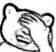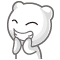# 博客

## 统计下现在有多少UOJ？

2018-05-10 15:18:49 By vfleaking

Hi，大家好，大家还记得我吗？

## 震惊！UOJ 近期出现一些 RE 的原因竟然是这个！

2017-05-01 23:56:24 By vfleaking## 关于最近 UOJ 遭受的 DDos 攻击

2017-02-04 15:04:01 By vfleaking

………………我也没辙啊

UPD： 3 次。

UPD： 4 次。（2017年2月8日）

UPD： 5 次。（2017年2月9日）

## Goodbye Bingshen 题解

2017-01-26 18:25:44 By vfleaking

“一起AK吧”

from nneztlk

### 直径拆除鸡

from szy042

（我没什么想说的

#### 算法三

UOJ的评测机很萌，所以能比算法一多十分~

### 快乐游戏鸡

from scPointer

#### 算法五

##### 算法5-1### 数据分块鸡

from jiry_2，数据和题解 from cy

### 同构判定鸡

from ppfdd，具体想法 from vfleaking，题解也是 vfleaking 写的。

YY 一个图同构算法 → 不会卡 → 再 YY 一个图同构算法 → 还是不会 → 要不咸鱼一下爆搜看看效果如何 → 那不就从构造题变成爆搜题了，不清真 → 继续 YY……

#### 卡算法四

A：$6$ 个点，$i$ 与 $(i + 1) \bmod 6$ 连边，$i$ 与 $(i + 3) \bmod 6$ 连边。

B：$6$ 个点，$i$ 与 $(i + 2) \bmod 6$ 连边，$i$ 与 $(i + 3) \bmod 6$ 连边。

## Goodbye Bingshen

2017-01-21 23:08:44 By vfleaking

UPD： 比赛已经结束！

echo -n 比赛开始2016s后的第一个提交 | md5sum
3af40cb9a16abb337db27feca1c2c0c1

1. Dylan_Sun
3. sjj118
4. xyz2606
5. JOHNKRAM## UOJ Test Round #2

2017-01-01 21:33:07 By vfleaking

UOJ Test Round #2 将于 1 月 8 日星期日晚上 19：00 举行！比赛将进行 3 个小时，共三道题。（咳咳大家这次在切题的时候一定要记得调戏下比赛问答系统，可以对题意不清的地方提问，出题人会进行回答哒）

（其实感觉这次难度确实好水好水，大家捉得开心就好）

UPD： 比赛已经结束！

echo -n 比赛中最后一个提问的 | md5sum
5d1ee77c1c495a6303e781cbe002b61b

1. Rui
3. xumingkuan
4. 613
5. philipsweng

## UOJ 开……开……开源了！

2016-10-03 00:59:02 By vfleakinghttps://github.com/vfleaking/uoj（咳咳 UOJ 用户群里的某些人应该改群名片了）

## UOJ Round #15 题解

2016-08-13 22:08:14 By vfleaking

from qmqmqm

### 奥林匹克环城马拉松

from ftiasch，题解 from C_SUNSHINE

#### 算法三

$$T \cdot \prod (deg_x-1)!$$

### 奥林匹克数据结构

from ppfdd，题解 from matthew99

## C/C++ 编译器更新到 4.8.4

2016-08-08 03:35:28 By vfleaking

（其实之前用在清华集训、清华校赛、清华夏令营的那版校内 UOJ 的编译器就是 4.8.4 的，不知大家注意到没有）

## 旷野大标程

2016-07-29 14:02:04 By vfleaking

#include "testlib.h"
#include <iostream>
#include <iomanip>
#include <vector>
#include <cassert>
using namespace std;

typedef long long int64;

int n = 0;

{
printf("+ %d %d\n", i, j);
return ++n;
}
int opneg(int i)
{
printf("- %d\n", i);
return ++n;
}
{
printf("C %d %s\n", i, c.c_str());
return ++n;
}
int opshl(int i, int k)
{
printf("< %d %d\n", i, k);
return ++n;
}
int opshr(int i, int k)
{
printf("> %d %d\n", i, k);
return ++n;
}
int opsig(int i)
{
printf("S %d\n", i);
return ++n;
}

int n_in = 0;
int opin()
{
printf("I\n");
return ++n;
}
int opout(int i)
{
printf("O %d\n", i);
return ++n;
}

struct xvar
{
int id;

xvar() {}
xvar(const int &_id)
: id(_id) {}

friend inline xvar operator+(const xvar &lhs, const string &rhs)
{
}
friend inline xvar operator+(const xvar &lhs, const xvar &rhs)
{
}
friend inline xvar operator<<(const xvar &lhs, const int &rhs)
{
return opshl(lhs.id, rhs);
}
friend inline xvar operator>>(const xvar &lhs, const int &rhs)
{
return opshr(lhs.id, rhs);
}
inline xvar operator-() const
{
return opneg(id);
}
};

inline xvar sig(xvar x)
{
return opsig(x.id);
}
inline xvar opout(xvar x)
{
opout(x.id);
return x;
}

inline string zeros(int n)
{
string s;
for (int i = 1; i <= n; i++)
s += '0';
return s;
}
inline string nines(int n)
{
string s;
for (int i = 1; i <= n; i++)
s += '9';
return s;
}

xvar psgn(xvar x)
{
return sig(x << 500);
}
xvar ge0(xvar x)
{
return psgn(x + ("0." + zeros(29) + "1"));
}
xvar ge1(xvar x)
{
return psgn(x + ("-0." + nines(30)));
}
xvar gt1(xvar x)
{
return psgn(x + ("-1." + zeros(29) + "1"));
}

xvar ncondx(xvar p, xvar x)
{
const int VL = 150;
p = p << (VL + 1);
xvar y = sig((x >> VL) + p);
return ((y + "-0.5") << (VL + 2)) + (-p);
}
xvar condx(xvar p, xvar x)
{
return ncondx(-p + "1", x);
}
xvar minx0(xvar x)
{
return ncondx(ge0(x), x);
}
xvar abs(xvar x)
{
const int VL = 150;
xvar p = ge0(x) << (VL + 2);
xvar y = sig((x >> VL) + p);
return x + ((-y + "0.5") << (VL + 3)) + p;
}

xvar land(xvar x, xvar y)
{
return gt1(x + y);
}
xvar lor(xvar x, xvar y)
{
return ge1(x + y);
}

xvar bits_to_x(xvar *bits)
{
xvar x = bits;
for (int i = 1; i < 32; i++)
x = (x << 1) + bits[i];
return x;
}
void x_to_bits(xvar x, xvar *bits)
{
x = x << 500;

long long t = 702955280397374434ll;
char ts;
for (int i = 31; i >= 1; i--)
{
sprintf(ts, "-%lld", t);
bits[i] = sig(x + (ts + zeros(142)));
x = x + -(bits[i] << (500 + i));
t /= 2;
}
bits = x >> 500;
}

xvar sqr(xvar x)
{
const int UL = 130;
xvar dx = x >> UL;
xvar y = sig(dx + "-1.098612288668109691395245236922525704647490557822749451734694333637494293218608966873615754");
y = y + -((dx >> 4) + (dx >> 3));

//   1.31695789692481670862504634730796844402698197146751647976847225692046018541644397607421903
// - 0.25
// = 1.06695789692481670862504634730796844402698197146751647976847225692046018541644397607421903

return (sig(y + "1.06695789692481670862504634730796844402698197146751647976847225692046018541644397607421903") + "-0.788675134594812882254574390250978727823800875635063438009301163241988836151466672846857700") << (UL * 2 + 7);
}

void solve1()
{
xvar x1 = opin();
xvar x2 = opin();
opout(-((x1 + x2) << 1));
}
void solve2()
{
xvar x = opin();
opout(sig(-(x + (x << 4))));
}
void solve3()
{
opout((psgn(opin()) << 1) + "-1");
}
void solve4()
{
xvar x = opin();
opout(abs(x));
}
void solve5()
{
static xvar bits;
for (int i = 0; i < 32; i++)
bits[i] = opin();
opout(bits_to_x(bits));
}
void solve6()
{
xvar x = opin();
static xvar bits;
x_to_bits(x, bits);
for (int i = 31; i >= 0; i--)
opout(bits[i]);
}
void solve7()
{
xvar x = opin();
xvar y = opin();
xvar xbits, ybits;
x_to_bits(x, xbits);
x_to_bits(y, ybits);

xvar zbits;
for (int i = 0; i < 32; i++)
{
xvar s = xbits[i] + ybits[i];
zbits[31 - i] = s + -(psgn(s + "-1.5") << 1);
}
opout(bits_to_x(zbits));
}
void solve8()
{
xvar x = opin();
string alpha = "2.06343706889556054705";
string sigalpha = "0.887298334620741688550198422716226773933599412474263948504833186119911079463425709202638465";
opout((sig((x >> 200) + alpha) + ("-" + sigalpha)) << 200);
}

void solve9()
{
xvar x;
for (int i = 1; i <= 16; i++)
x[i] = opin();
for (int i = 1; i <= 16; i++)
for (int j = i + 1; j <= 16; j++)
{
xvar s = x[i] + x[j];
x[i] = x[i] + minx0(-x[i] + x[j]);
x[j] = s + -x[i];
}
for (int i = 1; i <= 16; i++)
opout(x[i]);
}

xvar modadd(xvar a, xvar b, xvar ma, xvar negm)
{
xvar s = a + b;
return s + ncondx(psgn(-s + ma), negm);
}
xvar modmul(xvar a, xvar b, xvar m, xvar negm)
{
xvar ta;
xvar tb;
x_to_bits(a, ta);
x_to_bits(b, tb);

xvar zero = a >> 1000;
xvar ma = m + "-0.1";
a = ta;
a = a + ncondx(psgn(-a + ma), negm);
for (int i = 30; i >= 0; i--)
{
a = a + a + ta[i];
a = a + ncondx(psgn(-a + ma), negm);
}

xvar res = zero;
res = condx(tb, a);
res = res + ncondx(psgn(-res + ma), negm);

a = a + a;
a = a + ncondx(psgn(-a + ma), negm);
for (int i = 1; i < 32; i++)
{
res = res + condx(tb[i], a);
res = res + ncondx(psgn(-res + ma), negm);

if (i < 31)
{
a = a + a;
a = a + ncondx(psgn(-a + ma), negm);
}
}
return res;
}

xvar fastmodmul(xvar a, xvar b, xvar m)
{
xvar ab = sqr(a + b);
ab = ab + -sqr(a);
ab = ab + -sqr(b);
ab = ab + "0.2";
ab = -ab;
ab = ab << 149;
for (int i = 63; i >= 0; i--)
{
const int VL = 300;

xvar mi = m << (150 + i);
xvar p = sig(mi + ab) << (VL + 1);
xvar y = sig((mi >> VL) + p);
ab = ab + ((y + "-0.5") << (VL + 2)) + -p;
}
ab = ab >> 150;
ab = -ab + "-0.1";
return ab;
}

void solve10()
{
xvar a = opin(), b = opin(), m = opin();
//opout(modmul(a, b, m, -m));
opout(fastmodmul(a, b, m));
}

int main(int argc, char **argv)
{
freopen("nodes1.out", "w", stdout);
n = 0, solve1();
freopen("nodes2.out", "w", stdout);
n = 0, solve2();
freopen("nodes3.out", "w", stdout);
n = 0, solve3();
freopen("nodes4.out", "w", stdout);
n = 0, solve4();
freopen("nodes5.out", "w", stdout);
n = 0, solve5();
freopen("nodes6.out", "w", stdout);
n = 0, solve6();
freopen("nodes7.out", "w", stdout);
n = 0, solve7();
freopen("nodes8.out", "w", stdout);
n = 0, solve8();
freopen("nodes9.out", "w", stdout);
n = 0, solve9();
freopen("nodes10.out", "w", stdout);
n = 0, solve10();

return 0;
}